# ALE框架下虚拟流体方法模拟移动边界的一维可压缩多介质流动问题GFM for One-Dimensional Compressible Multi-Material Flows with Moving Boundary on ALE Frame

• 全文下载: PDF(887KB)    PP.1638-16440   DOI: 10.12677/AAM.2018.712191
• 下载量: 180  浏览量: 282

We use the ghost ﬂuid method to deal with the moving boundary one-dimensional compressible multi-material ﬂows problem on ALE schemes. The grids at the boundary move along the boundary to keep track the moving boundary while the inner grids are adjusted continuously to improve the over-all grid quality. Trace the material interface using Level set equation on ALE schemes. Transform the multi-material ﬂows problem into single-medium problems to avoid non-physical oscillations near the material interface through ghost ﬂuid.

1. 引言

ALE方法是由Hirt  等首先提出。ALE方法具有任意网格移动速度，具有良好的灵活性和适用性，可以处理复杂边界和移动边界的问题。良好的网格移动策略，既可以避免大变形带来的网格严重扭曲变形问题。

2. 理论方法

2.1. 控制方程

$\frac{\partial U}{\partial t}+\frac{\partial F\left(U\right)}{\partial x}=0,$ (2.1)

$U=\left[\begin{array}{c}\rho \\ \rho u\\ \rho E\end{array}\right],\text{\hspace{0.17em}}\text{\hspace{0.17em}}\text{\hspace{0.17em}}F\left(U\right)=\left[\begin{array}{c}\rho u\\ \rho {u}^{2}+p\\ \rho Eu+pu\end{array}\right],$

$E=e+\frac{{u}^{2}}{2}$ (2.2)

$p=\left(\gamma -1\right)\rho e$

2.2. ALE方法数值离散

${\int }_{\Omega \left(t\right)}\frac{\partial U}{\partial t}\text{d}V+{\int }_{\Omega \left(t\right)}\left(\frac{\partial F}{\partial x}+\frac{\partial G}{\partial y}\right)\text{d}V=0.$ (2.3)

$\frac{\text{d}}{\text{d}t}{\int }_{\Omega \left(t\right)}U\text{d}V+{\int }_{\partial \Omega \left(t\right)}\left(\left(F,G\right)\cdot N-\left(w\cdot N\right)U\right)\text{d}l=0.$ (2.4)

${U}_{i}^{n+1}=\frac{\Delta {x}_{i}^{n}}{\Delta {x}_{i}^{n+1}}{U}_{i}^{n}-\frac{\Delta t}{\Delta {x}_{i}^{n+1}}\left({F}_{i+\frac{1}{2}}-{F}_{i-\frac{1}{2}}+{w}_{i-\frac{1}{2}}{U}_{i-\frac{1}{2}}-{w}_{i+\frac{1}{2}}{U}_{i+\frac{1}{2}}\right)$ (2.5)

$\begin{array}{l}{U}_{t}+{F}_{x}=0,\hfill \\ IVS:\text{\hspace{0.17em}}\text{\hspace{0.17em}}U\left(x,0\right)=\left\{\begin{array}{l}{U}_{L}={U}_{i}^{n},\text{\hspace{0.17em}}\text{\hspace{0.17em}}\text{\hspace{0.17em}}\text{\hspace{0.17em}}x<0,\\ {U}_{R}={U}_{i+1}^{n},\text{\hspace{0.17em}}\text{\hspace{0.17em}}\text{\hspace{0.17em}}x>0.\end{array}\hfill \end{array}$ (2.6)

${F}_{i+\frac{1}{2}}-{w}_{i+\frac{1}{2}}{U}_{i+\frac{1}{2}}={F}^{{w}_{i+\frac{1}{2}}}-{w}_{i+\frac{1}{2}}{U}^{{w}_{i+\frac{1}{2}}}$ (2.7)

2.3. Level Set方程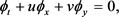(2.8)

${\varphi }_{t}+S\left({\varphi }_{0}\right)\left(\sqrt{{\varphi }_{x}^{2}+{\varphi }_{y}^{2}}-1\right)=0$ (2.9)

2.4. Ghost Fluid方法

Ghost Fluid方法很好的结合了上述两种方法的优点，利用Level Set追踪物质界面，然后通过虚拟流体，将一个多介质问题转化为多个单介质问题，再通过求解单介质的方法来分别求解每种流体，这样可以成功避开界面两侧流体由于状态方程不同而带来的振荡问题。同时本文采用ALE方法，不断地调整网格质量，从而避免了网格严重变形的问题。虚拟流体的具体实施过程参考  ，如图1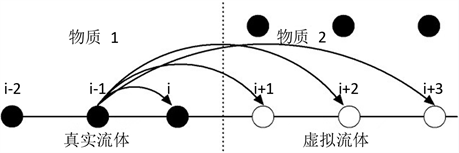Figure 1. Ghost Fulid

3. 移动边界和网格移动速度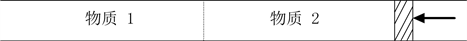Figure 2. Shock tube with a moving piston

${x}_{i-\frac{1}{2}}^{old}=a+\Delta x\left(i-1\right),\text{\hspace{0.17em}}\text{\hspace{0.17em}}\text{\hspace{0.17em}}\text{\hspace{0.17em}}i=1,2,\cdots ,N+1$ (3.1)

${x}_{piston}^{new}=b+{\int }_{0}^{\Delta t}{u}_{piston}\left(t\right)\text{d}t$ (3.2)

${x}_{i-\frac{1}{2}}^{new}=a+\frac{{x}_{i-\frac{1}{2}}^{new}-{x}_{i-\frac{1}{2}}^{old}}{\Delta t},\text{\hspace{0.17em}}\text{\hspace{0.17em}}\text{\hspace{0.17em}}i=1,2,\cdots ,N+1$ (3.3)

${w}_{i-\frac{1}{2}}=\frac{{x}_{i-\frac{1}{2}}^{new}-{x}_{i-\frac{1}{2}}^{old}}{\Delta t},\text{\hspace{0.17em}}\text{\hspace{0.17em}}\text{\hspace{0.17em}}\text{\hspace{0.17em}}i=1,2,\cdots ,N+1$ (3.4)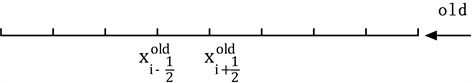(a)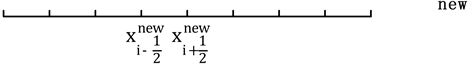(b)

Figure 3. The old and new cells for ALE

4. 数值算例

$\left\{\begin{array}{l}\rho =1.0,\text{\hspace{0.17em}}\text{\hspace{0.17em}}u=0.75,\text{\hspace{0.17em}}\text{\hspace{0.17em}}p=1.0,\text{\hspace{0.17em}}\text{\hspace{0.17em}}\gamma =1.4,\text{\hspace{0.17em}}\text{\hspace{0.17em}}\text{\hspace{0.17em}}\text{\hspace{0.17em}}\text{ }\text{\hspace{0.17em}}\text{ }\text{ }x<0.25,\\ \rho =0.125,\text{\hspace{0.17em}}\text{\hspace{0.17em}}u=0.0,\text{\hspace{0.17em}}\text{\hspace{0.17em}}p=0.1,\text{\hspace{0.17em}}\text{\hspace{0.17em}}\gamma =1.2,\text{\hspace{0.17em}}\text{\hspace{0.17em}}\text{\hspace{0.17em}}\text{\hspace{0.17em}}x>0.25.\end{array}$ (4.1)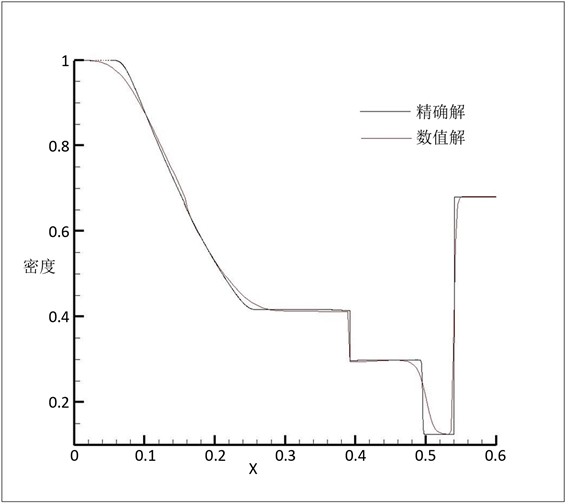Figure 4. Density for exact results and numerical results

5. 结论

  Benson, D. (1992) Computational Methods in Lagrangian and Eulerian Hydrocodes. Computer Methods in Applied Mechanics and Engineering, 99, 235. https://doi.org/10.1016/0045-7825(92)90042-I  Fedkiw, R.P., Aslam, T., Merriman, B. and Osher, S. (1999) A Non-Oscillatory Eulerian Approach to Interfaces in Multimaterial Flows (the Ghost Fluid Method). Journal of Computational Physics, 152, 457-492. https://doi.org/10.1006/jcph.1999.6236  Hirt, C.W., Amsden, A.A. and Cook, J.L. (1997) An Arbitrary La-grangian-Eulerian Computing Method for All Flow Speeds. Journal of Computational Physics, 135, 203-216. https://doi.org/10.1006/jcph.1997.5702  Toro, E.F. Riemann Solvers and Numerical Methods for Fluid Dy-namics: A Practical Introduction. Riemann Solvers and Numerical Methods for Fluid Dynamics.  Shen, Z., Yan, W. and Yuan, G. (2014) A Robust and Contact Resolving Riemann Solver on Unstructured Mesh, Part I I, ALE Method. Journal of Computational Physics, 268, 456-484. https://doi.org/10.1016/j.jcp.2014.03.003